# 经纬度距离计算及优化方案

## 不规则的地球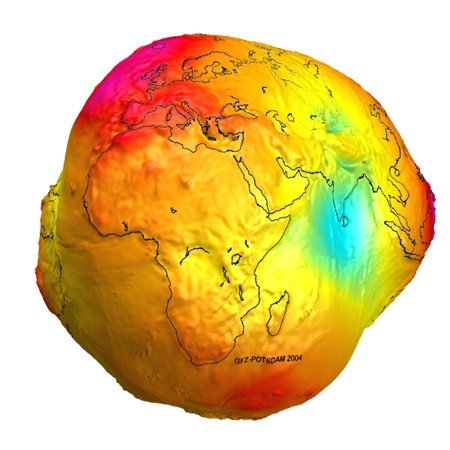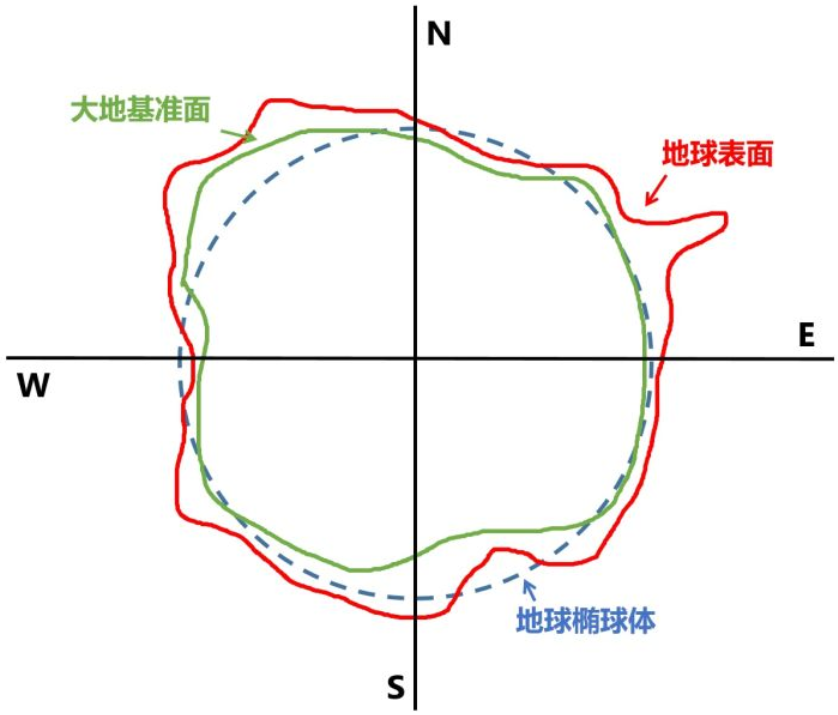## 经纬度小数位数对精度的影响

• 经度每隔0.000001度，距离相差约1米
• 经度每隔0.00001度，距离相差约10米
• 经度每隔0.0001度，距离相差约100米
• 经度每隔0.001度，距离相差约1000米
• 经度每隔0.01度，距离相差约10000米

• 纬度每隔0.000001度，距离相差约1.1米
• 纬度每隔0.00001度，距离相差约11米
• 纬度每隔0.0001度，距离相差约111米
• 纬度每隔0.001度，距离相差约1113米
• 纬度每隔0.01度，距离相差约11132米

• 地球的赤道半径 = 6378.1 公里
• 地球的极半径 = 6356.8 公里
• Latitude的范围是：-90 到 +90
• Longitude的范围：-180 到 +180
• 地球参考球体的周长：68米• 纬度每格1度影响的距离 = 极半径*π/180=110.95 km
• 经度每隔1度影响的距离（赤道）= 赤道半径*π/180 = 111.32 km，维度越靠近南北极，影响的距离越小。

## 经纬度距离计算方法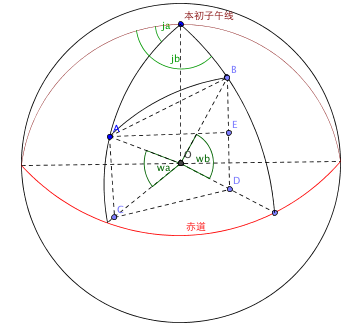$$S = 2 \times \arcsin(\sqrt{\sin^2(\frac{a}{2})+\cos(Lat1)\times \cos(lat2) \times sin^2(\frac{b}{2})}) \times 6378.137$$

private const double EARTH_RADIUS = 6378.137;

{
return d * Math.PI / 180.0;
}

public static double GetDistance(double lat1, double lng1, double lat2, double lng2)
{
s = Math.Round(s * 10000) / 10000;
return s;
}


var j = void 0,
p = null,
H.prototype.nb = function(a) {
return a && this.lat == a.lat && this.lng == a.lng
};

getDistance: function(a, b) {
if (a && b) {
if (a.nb(b)) return 0;
var c = 0,
c = R.To(a, b);
if (c === p || c === j) c = 0;
return c
}
},

nb: function(a) {
return !(a instanceof eb) || this.xj() ? q : this.se().nb(a.se()) && this.of().nb(a.of())
},

function R() {}
R.prototype = new gc;
x.extend(R, {
To: function(a, b) {
if (!a || !b) return 0;
a.lng = this.ND(a.lng, -180, 180);
a.lat = this.RD(a.lat, -74, 74);
b.lng = this.ND(b.lng, -180, 180);
b.lat = this.RD(b.lat, -74, 74);
return this.Re(this.Sk(a.lng), this.Sk(b.lng), this.Sk(a.lat), this.Sk(b.lat))
},
Re: function(a, b, c, d) {
return this.jP * Math.acos(Math.sin(c) * Math.sin(d) + Math.cos(c) * Math.cos(d) * Math.cos(b - a))
},
jP: 6370996.81,

RD: function(a, b, c) {
b != p && (a = Math.max(a, b));
c != p && (a = Math.min(a, c));
return a
},
ND: function(a, b, c) {
for (; a > c;) a -= c - b;
for (; a < b;) a += c - b;
return a
}
Sk: function(a) {
return Math.PI * a / 180
},
});


def get_distance_bd09(point_a, point_b):
"""
算法来源：http://developer.baidu.com/map/jsdemo.htm#a6_1
:param pointA: {lat:29.490295, lng:106.486654}
:param pointB: {lat:29.615467, lng:106.581515}
:return:米
"""
R = 6370996.81 #球半径

if (point_a and point_b):
if point_a["lat"] == point_b["lat"] and point_a["lng"] == point_b["lng"]:
distance = 0
else:
a_lat = point_a["lat"] * math.pi / 180
a_lng = point_a["lng"] * math.pi / 180
b_lat = point_b["lat"] * math.pi / 180
b_lng = point_b["lng"] * math.pi / 180
# print(a_lng,b_lng,a_lat,b_lat)
distance = R * math.acos(math.sin(a_lat) * math.sin(b_lat) + math.cos(a_lat) * math.cos(b_lat) * math.cos(b_lng - a_lng))
return distance


from math import radians, sin, cos, asin, sqrt

def haversine(latlon1, latlon2):
lat1, lon1 = latlon1
lat2, lon2 = latlon2
lon1, lat1, lon2, lat2 = map(radians, [lon1, lat1, lon2, lat2])
dlon = lon2 - lon1
dlat = lat2 - lat1
a = sin(dlat / 2) ** 2 + cos(lat1) * cos(lat2) * sin(dlon / 2) ** 2
c = 2 * asin(sqrt(a))
r = 6370996.81 #球半径
return c * r


USE [testdb]
GO
SET ANSI_NULLS ON
GO
SET QUOTED_IDENTIFIER ON
GO
CREATE FUNCTION [dbo].[f_GetDistance]
(
@GPSLng DECIMAL(12,6),
@GPSLat DECIMAL(12,6),
@Lng  DECIMAL(12,6),
@Lat DECIMAL(12,6)
)
RETURNS DECIMAL(12,4)
AS
BEGIN
DECLARE @result DECIMAL(12,4)
IF @GPSLng = @Lng AND @GPSLat = @Lat
BEGIN
SELECT @result = 0
END
ELSE
BEGIN
SELECT @result = 6370996.81*ACOS(SIN(@GPSLat/180*PI())*SIN(@Lat/180*PI())+COS(@GPSLat/180*PI())*COS(@Lat/180*PI())*COS((@GPSLng-@Lng)/180*PI()))
END
RETURN @result
END


## 经纬度距离计算优化

### 方案二：Uber H3法

• Uber H3网格精度更高一些
• Uber H3为六边形网格，更接近圆
• Uber H3提供Ring方法，可以按照设定的K值获取中心网格周边距离第K个网格。

### 方案三：经纬度精度值限制法

• 纬度每格1度影响的距离 = 极半径*π/180=110.95 km
• 经度每隔1度影响的距离（赤道）= 赤道半径*π/180 = 111.32 km，维度越靠近南北极，影响的距离越小。

import pandas as pd

df = pd.DataFrame([{'item_id': 1234, 'lat': 39.93607, 'lon': 116.42394},
{'item_id': 1235, 'lat': 39.93526, 'lon': 116.42590},
{'item_id': 1236, 'lat': 39.92969, 'lon': 116.42961}])
df['tmp'] = 1
df = pd.merge(df, df, on='tmp')
df = df[df.item_id_x != df.item_id_y]
df = df[(abs(df['lat_x'] - df['lat_y']) < 0.02) & (abs(df['lng_x'] - df['lng_y']) < 0.02)]


### 方案四：简化经纬度计算公式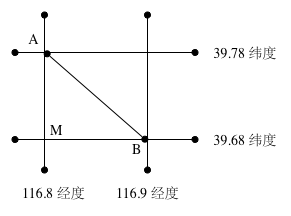from math import radians, cos, sqrt

def haversine_hack(lat1, lon1, lat2, lon2):
lon1, lat1, lon2, lat2 = map(radians, [lon1, lat1, lon2, lat2])
dx = lon2 - lon1  # 经度差值
dy = lat2 - lat1  # 纬度差值
b = (lat1 + lat2) / 2  # 平均纬度
r = 6370996.81  # 球半径
lx = dx * r * cos(b)  # 东西距离
ly = dy * r  # 南北距离
return sqrt(lx ** 2 + ly ** 2)


df['dist'] = df.apply(lambda row: haversine(row['lat_x'], row['lon_x'], row['lat_y'], row['lon_y']), axis=1)
df['dist_hack'] = df.apply(lambda row: haversine_hack(row['lat_x'], row['lon_x'], row['lat_y'], row['lon_y']), axis=1)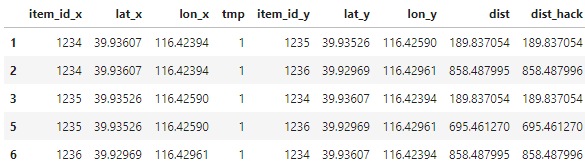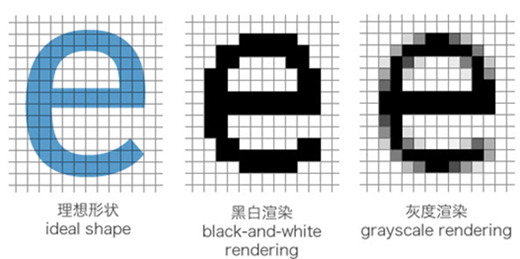##### 网站与APP开发中的字体设置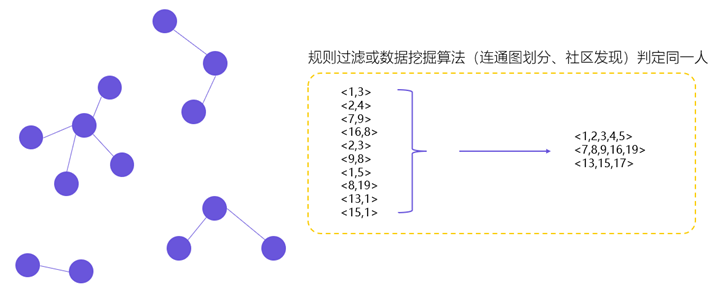##### 用户体系搭建之ID-Mapping